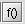# Current value of an I/O parameter

## Introduction

To recover a current value (value for a computation step):

• of an I/O parameter
• of a sensor
• of a kinematic quantity
• of a circuit quantity

the user can use the command Current value of an I/O parameter.

## Current value of an I/O parameter

To recover a current value, follow instructions below:

Step Action
1 In the menu Advanced point on Current value of an I/O parameter
2

In the dialog box Current value of an I/O parameter:

• at the end of the formula zone, click on3

In the dialog box Editor of formula and I/O parameters

choose:

• in the Geometric tab: a geometric parameter or edit a formula

• in the Physical tab: an I/O parameter or edit a formula

• in the Sensor tab: a sensor or edit a formula

• in the Mechanical set tab: kinematic characteristics

• in the Circuit tab: electrical characteristics

4 Modify the name of the default result (optional)

The result is displayed in the History zone and is stored in the data tree.

## Equivalent commands

The sensors and the I/O parameters have each a command equivalent to the Current value of an I/O parameter command:

Type of parameters Equivalent command
Sensor Create a result with current value of sensor
I/O Parameter Create a result with a current value of an I/O parameter

These commands are available in the contextual menu specific for various sensors and I/O parameters.

## Storage

The result is stored as an entity in the tree of the general data in the type of entity Result under the root of the Post processing repertory.

If the calculation cannot be carried out for the resolution step chosen, the value is set to zero and an indicator informs the user: invalid result.